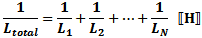Please Support RF Cafe by purchasing my  ridiculously low−priced products, all of which I created.

RF Workbench  (shareware)# Resistors and Resistance CalculationsResistors are passive devices used in electronic circuits to control the flow of current. An ideal resistor has no associated stray capacitance or inductance, and therefore performs exactly the same in a direct current (DC) circuit as it does in an alternating current (AC) circuit at any frequency.

 Calculating Resistance from Bulk ResistivityAs an example of calculating a volume's resistance from its bulk resistivity, consider the drawing to the left. Assume 12 AWG copper wire with a resistivity (from the table) of 1.72x10-6 Ω·cm, a cross-sectional area (A) of 0.03309 cm2, and a length of 1 meter. Per the given formula, its resistance is: which agrees well with typical specified Ω/km values published by wire manufacturers. Alpha claims 1.59 Ω/1000' or 5.22 Ω/km.
Real-world resistors made of physical components exhibit more than just a pure resistance when present in an AC circuit. A common circuit simulator model is shown to the left. It includes the actual ideal resistor with a parallel capacitive component representing inter-element (if present) mutual capacitance, and a series inductive component representing lead and/or solder/bond pads that respond to alternating current. SPICE-type simulators use this or an even more sophisticated model to facilitate more accurate calculations over a wide range of frequencies.

Equations (formulas) for combining inductors in series and parallel are given below.

 Parallel-Connected ResistorsTotal resistance of parallel-connected resistors is equal to the reciprocal of the sum of the reciprocals of the individual resistances. Keep units constant. Series-Connected ResistorsTotal resistance of series-connected resistors is equal to the sum of the individual resistances. Keep units constant. Calculating Resistance from Bulk ResistivityAs an example of calculating a volume's resistance from its bulk resistivity, consider the drawing to the left. Assume 12 AWG copper wire with a resistivity (from the table) of 1.72x10-6 Ω·cm, a cross-sectional area (A) of 0.03309 cm2, and a length of 1 meter. Per the given formula, its resistance is: